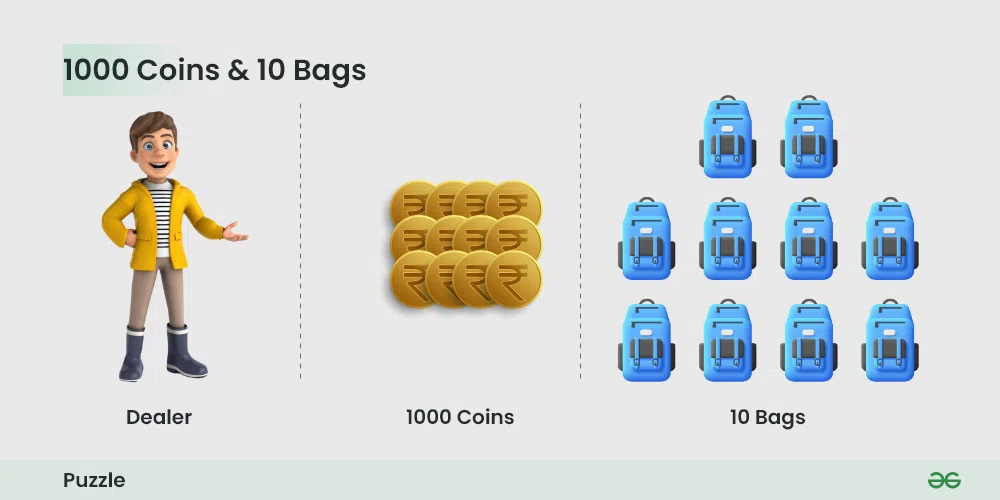# Puzzle 11 | ( 1000 Coins and 10 Bags )

A dealer has 1000 coins and 10 bags. He has to divide the coins over the ten bags so that he can make any number of coins simply by handing over a few bags. How must divide his money into the ten bags?Find the ages of daughters

Solution:

The idea of filling coins in the powers of 2 can be get from the condition that was given the question that each bag can be given or not given: we are not allowed to open the bag and give some coins. So we can represent each bag as each place value which takes either the value of 0 or 1.

Example:

If we have to give 7 coins then it is nothing but 0000000111 the last 3 digits of 1 represent that those 3 bags whose place values are 1,2,4 are to be given to give 7 coins
Remember that each bag should be either given or not given, which means there are only 2 choices to us, which is similar to the numbers in base 2 where each digit has only 2 values either 1 or 0.
We can fill coins in the 10 bags in increasing order of 2^n where n varies from 0 – 8, filling the last bag with all remaining coins as follows:

1 = 2^0 = 1
2 = 2^1 = 2
3 = 2^2 = 4
4 = 2^3 = 8
5 = 2^4 = 16
6 = 2^5 = 32
7 = 2^6 = 64
8 = 2^7 = 128
9 = 2^8 = 256
10 = remaining coins = 489

Now, the dealer can make any number of coins just by handing over the bags.

As,
number of coins needed = some bag 1 + some bag 2 + ….. + some bag n
example:
519 coins = bag 2 + bag 4 + bag 8 + bag 16 + bag 489

Factorization algorithms other than powers of 2 are costly on computer systems. Please share any other information. Any person working on cryptography can share more details.

Whether you're preparing for your first job interview or aiming to upskill in this ever-evolving tech landscape, GeeksforGeeks Courses are your key to success. We provide top-quality content at affordable prices, all geared towards accelerating your growth in a time-bound manner. Join the millions we've already empowered, and we're here to do the same for you. Don't miss out - check it out now!